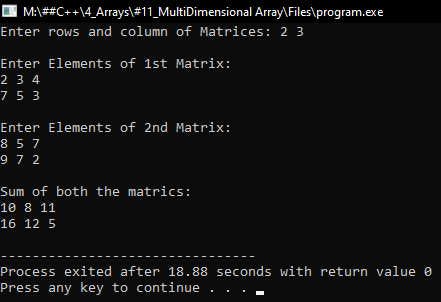# Multidimensional Array in C++

## Multidimensional Array in C++ with Examples:

In this article, I am going to discuss Multidimensional Array in C++ Language with examples. Please read our previous articles, where we discussed Drawing Patterns using Nested Loops in C++ Language with examples. Almost all programming languages support multi-dimensional arrays and C++ also supports Multidimensional Arrays. We have already seen the single-dimensional Array. Commonly we study single dimension and two-dimensional arrays.

##### Different Types of an Array in C++:

Different types of arrays in C++ are as follows.

1. One dimensional array
2. Two-dimensional array
3. Multi-dimensional array

One-dimensional array: How to declare, initialize and access a one-dimensional array is already discussed in our previous articles. That means as of now what we discussed is a one-dimensional array.

###### Two-dimensional array:

A two-dimensional array is an array in which each element is referred by two indexes. Element in the 2D array is stored in the matrix form. The first index shows the row of the matrix and the second index shows the column of the matrix.

Example: int matrix;

2D Array in Memory is shown below to access elements in the zeroth index we need to specify two indexes matrix.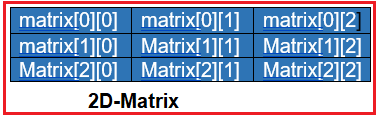##### How 2D Array is Created and Accessed in C++?

The method for creating a two-dimensional array is,

int A;

If we created like this, we imagine that the array is created with 3 rows and 4 columns where the name of an array is ‘A’.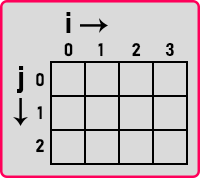‘j’ represents column number and ‘i’ represents row number. We can access any element with the row and column number as,

cout << A << endl;

This means 1st row and 2nd column.

Note: Indexing starts from 0 onwards in the array. So, we have started row and column from 0. This is how we can access any location. Addressing of 2d array is mapped same as single dimension array.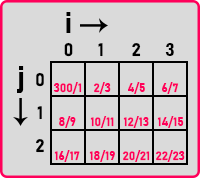Location is allocated continuously side by side. So basically, it will create a single dimension array of size 12, where the first four locations are used as first rows, the second four locations are used as the second row and the rest locations are used as the third row.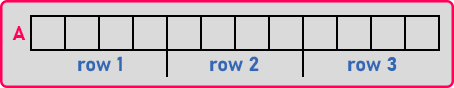But the compiler will allow us to access this single dimension array as a 2D array. Next, let us see how to create and initialize a 2D array.

##### Initializing a 2D array in C++:

int A = {{2, 5, 9},{6, 9, 15}};

This is declaration + initialization of a 2D array. Here 2,5,9 is the 1st row and 6,9,15 is the 2nd row. This is how they will be filled and we can access any element with the help of two indices that is row number and column number. Now, the other way of initializing it is,

int A = {2,5,9,6,5,15};

Even we can write as,

int A = {2,5 };

We have taken the same size and if we fill up only 2 values then the rest of the values will be initialized automatically by zero. So, this is how we can declare & initialized a 2D array. Next, let us see how to access the elements of the 2-D array.

##### Accessing the elements of the 2D array in C++:

For accessing all the elements basically, we require nested ‘for’ loop, one ‘for’ loop for the row, and another ‘for’ loop for columns.

```for(int i = 0; i < 2; i++){
for(int j = 0; j < 3; j++){
cout << A[i][j];
}
cout << endl;
}
```

This code will display all the elements of the 2D array. So, in this way, all the elements will be accessed. Now let us write some programs.

##### Program to print all the elements of the array:
```#include <iostream>
using namespace std;
int main()
{
int n, m;
cout << "Enter rows and column: ";
cin >> n >> m;
int A[n][m];
cout << "\nEnter Elements of Array:\n";
for (int i = 0; i < n; i++)
{
for (int j = 0; j < m; j++)
{
cin >> A[i][j];
}
}
cout << "\nElements are: \n";
for (int i = 0; i < 2; i++)
{
for (int j = 0; j < 3; j++)
{
cout << A[i][j] << " ";
}
cout << endl;
}
}
```
###### Output:##### Program to add 2 Matrices using C++:
```#include <iostream>
using namespace std;
int main()
{
int n, m;
cout << "Enter rows and column of Matrices: ";
cin >> n >> m;
int A[n][m], B[n][m], C[n][m];

cout << "\nEnter Elements of 1st Matrix:\n";
for (int i = 0; i < n; i++)
{
for (int j = 0; j < m; j++)
{
cin >> A[i][j];
}
}
cout << "\nEnter Elements of 2nd Matrix:\n";
for (int i = 0; i < n; i++)
{
for (int j = 0; j < m; j++)
{
cin >> B[i][j];
}
}
cout << "\nSum of both the matrics: \n";
for (int i = 0; i < 2; i++)
{
for (int j = 0; j < 3; j++)
{
C[i][j] = A[i][j] + B[i][j];
cout << C[i][j] << " ";
}
cout << endl;
}
}
```
###### Output: